Next: Orbit interpolation Up: Definitions Previous: pixel number   Contents

# Doppler, range and ellipsoid equations

The following three equations are used regularly throughout the software to compute the point P that corresponds to a certain line and pixel in the master or slave image (see also ). Precise orbits are necessary.

1. Doppler: The point P at the surface lies perpendicular to the orbit due to zero Doppler processing (otherwise this equation has to be adapted with a slant angle).
2. Range: The geometrical distance to P on the surface is equal to the speed of light times the range time.
3. Ellipsoid: Force the point to lie on an ellipsoid.

The equations for the point P on the ellipsoid and the satellite S in its orbit are (where x denotes (x,y,z)):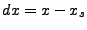(D43)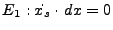(D44)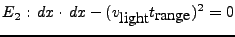(D45)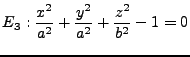(D46)

To compute the coordinates of a point P on the ellipsoid, corresponding with line l and pixel p in the master image the following has to be done. First the position of the satellite has to be computed (assumed exact) based on the line number and PRF (l to azimuth time to interpolated position), and the velocities for this time (by interpolation). Also the range time corresponding to the pixel number is computed (based on RSR, assumed exact).

Next the set of equations is used to solve for P(x,y,z). This is done iteratively by linearization, which requires the derivative of the equations to x and approximate values for the unknowns (the coordinates of the center (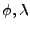) given in the SLC leader file, converted to xyz on a sphere).(D47)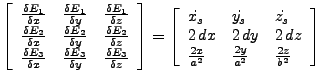(D48)

Solving this exactly determined system of 3 equations yields the next solution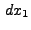and the new values for the unknowns become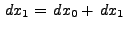which are used to computeand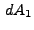. The solution is updated until convergence (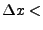1e-6 meters).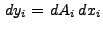(D49)

Where: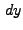contains the observations. (set of equations)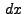contains the unknowns (coordinates of P).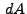contains the partials (evaluated for previous solution).

To solve for the azimuth time if the coordinates of a point on the ground is known, only the Doppler D.45 equation needs to be used. the derivative with respect to azimuth time of this equation equals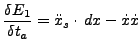(D50)

The solution is equal to (use approximate solution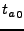to evaluate these expressions).(D51)

and(D52)

The solution is updated until convergence (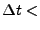1e-10 seconds).

The range time is then computed as in equation D.46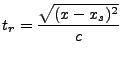(D53)Next: Orbit interpolation Up: Definitions Previous: pixel number   Contents
Leijen 2009-04-14